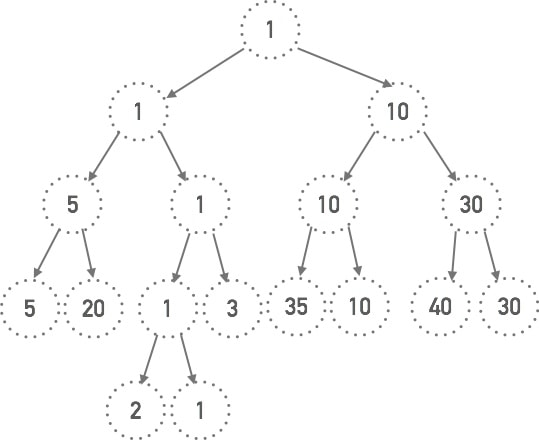# Tournament Tree

Posted by JH

## Second MIN in Tournament Tree

A tournament tree is a binary tree in which the parent is the minimum of the two children.
Given a tournament tree find the 2nd minimum value in the tree.
A node in the tree will always have 2 or 0 children.
All leaves have distinct and unique values.

# Solution

Where is the 2nd MIN? Let’s start from drawing a tournament tree and observe.Some features of a tournament tree:
I. Root always has the MIN value since the winner is the smaller number between the two participants.
II. An ancestous node is always greater or equal to a descendent one.
III. Based on II, we can tell that any nodes under the loser (root.right) of the final match (between root.left and root.right) will only be larger than or equal to loser. Since the loser (root.right.val) is greater than the winner (root.val), anything lower than the loser won’t be the second MIN value for sure.
Loser by itself can be the potential second MIN value if the winner side of the subtree is empty or has large values. So take loser of root as a candidate for second MIN value and ignore the rest of the loser side tree.
IV. Having sorted out the loser side, now let’s look at the winner side (root.left in the example). Root.left by itself is a tournament tree with the same winner as the main tree. Based on III, any nodes under the loser side (root.left.left) of root.left will not be the second MIN. So take loser of root.left as a candidate for second MIN and ignore the rest of the loser side.
So we again look at the winner side (root.left.right) which by itself is a tournament tree with the same winner as root. Therefore the root node on its loser side is a candidate as the second MIN, or the second MIN could reside in the winner side that takes further searching to be found.

Now it is clear that the same pattern applies to any layer of the subtrees.

Depth First Search Recursive function findSecondMIN(root) applies to ONLY the winner side of every subtree. When the second MIN of the winner side tree is figured out, compare it with the loser’s value and return the smaller one between the two. That will be the 2nd MIN of the root.

Base case: when to stop searching? At any leaf node, there is no second MIN value for a tree with no descendent. In this case return positive infinity or NULL to tell the upper layer of recursion, do not select the result from this side of the tree.

# Implementation

``````class Node {
Integer value;
Node left, right;
}

//If the tree has no 2nd min value, return -1
public class TournamentTree {
int secondMinValue(Node root) {
if(root == null || root.left == null) {
return -1;
}
if(root.left.value == root.value) {  //if root.left is winner
int leftSecondMin = secondMinValue(root.left);   //search for 2nd min at winner side
if(leftSecondMin == -1 || leftSecondMin > root.right.value) {
return root.right.value;
} else return leftSecondMin;
} else {   //if root.right is winner
int rightSecondMin = secondMinValue(root.right);
if(rightSecondMin == -1 || rightSecondMin > root.left.value) {
return root.left.value;
} else return rightSecondMin;
}
}
}
``````